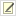# Mutation Rates from Genome Resequencing

Motivation: You have re-sequenced several genomes after a mutation accumulation or adaptive evolution experiment. How do you infer the rates at which different types of mutation accumulate from these data? What are the 95% confidence intervals on these values?

## Case 1: Mutations with many identical sites

Assumptions:

1. The number of mutations is small compared to the number of sites.
2. There are no back mutations (reversions).
3. Mutations rates are constant over time and across sites.

Example: Single-base substitutions

Calculation:

1. If you restrict your data to one genome per experimental population, then you can calculate the maximum likelihood value and 95% confidence limits from a Poisson distribution. Count the total number of mutations (m) and the total number of elapsed generations or time of independent evolution (T). Example: 22 point mutations found in 6 genomes that each evolved for 10,000 generations.
```>m = 22
>T = 10000 * 6
>rate = poisson.test(m)
>rate\$estimate/T
event rate
0.0003666667
>rate\$conf.int/T
 0.0002297880 0.0005551377
attr(,"conf.level")
 0.95
```
2. If you know the number of sites at risk for the mutation (s), then you can calculate a per-site mutation rate. Example: Assume these 22 point mutations are A to G substitutions and there are 1,342,726 A bases in the original genome.
```>s = 1342726
>rate\$estimate/(T*s)
event rate
2.730763e-10
>rate\$conf.int/(T*s)
 1.711355e-10 4.134408e-10
attr(,"conf.level")
 0.95
```

## Case 2: One-time mutations

Assumptions:

1. The mutation can only happen once per genome (e.g., deletion of a certain region).
2. The mutation rate is constant per unit time or generation.

Example: Deletion of an unstable chromosomal region. Once deleted, it can never be deleted again.

Calculation:

1. Count the number of independent genomes that have the mutation (m) and total number of genomes analyzed (n) at a given time (T). Example: 5 of 12 independently evolved genomes have the mutation after 10,000 generations.
```> m = 5
> n = 12
> T = 10000
```
2. Calculate a maximum likelihood value and 95% exact (Clopper-Pearson) confidence limit for the fraction of independently evolved lineages that do not have the mutation from your observations.
```p = binom.test(n - m, n)
>p

Exact binomial test

data:  n - m and n
number of successes = 7, number of trials = 12, p-value = 0.7744
alternative hypothesis: true probability of success is not equal to 0.5
95 percent confidence interval:
0.2766697 0.8483478
sample estimates:
probability of success
0.5833333
```
3. If the mutations happen at a constant rate per unit time, then you can calculate the rate that gives this fraction of independent lineages without a mutation up to the given time point using the zero event term from a Poisson process:
```> -log(p\$estimate) / T
probability of success
5.389965e-05
> -log(p\$conf.int) / T
 1.284931e-04 1.644646e-05
attr(,"conf.level")
 0.95
```

This is a particularly simple type of survival analysis.

## More complex situations

What if you want to test for variation in rates of mutation accumulation?

You can use Poisson regression in R (using `glm()`) to judge whether there is a significant difference in the rates at which mutations accumulate relative to some factor. For example, you can test whether there is evidence that certain populations accumulated different numbers of mutations per unit time compared to others or whether mutations at certain sites were more common than at other sites. Fit a model that incorporates the relevant factor and one that does not, and then compare them using `anova()`.

What if you sequenced multiple genomes from each population?

This type of pseudo-replication complicates the statistical analysis because strains sequenced from one population are likely to share some of their evolutionary history. If they happened to evolve more rapidly by chance, you will overestimate rates by including both of them and assuming an independent time basis for each one. It is not easy to correct for this shared history. To do so in a rigorous way would likely require a resampling procedure. It would be valid to randomly pick one strain from each population and only include that one in the typical analysisrestoring the assumption of independencebut this is excluding some information.

## Reference

We used the approaches described here to characterize and compare the rates of mutations in this paper:

Renda, B.A., Dasgupta, A., Leon, D., Barrick, J.E. (2015) Genome instability mediates the loss of key traits by Acinetobacter baylyi ADP1 during laboratory evolution. J. Bacteriol. 197:872-881. https://doi.org/10.1128/JB.02263-14Barrick Lab  >  ProtocolList  >  ProceduresCalculatingMutationRatesFromGenomicData

Contributors to this topicJeffreyBarrick
Topic revision: r4 - 2020-10-31 - 14:42:10 - Main.JeffreyBarrick